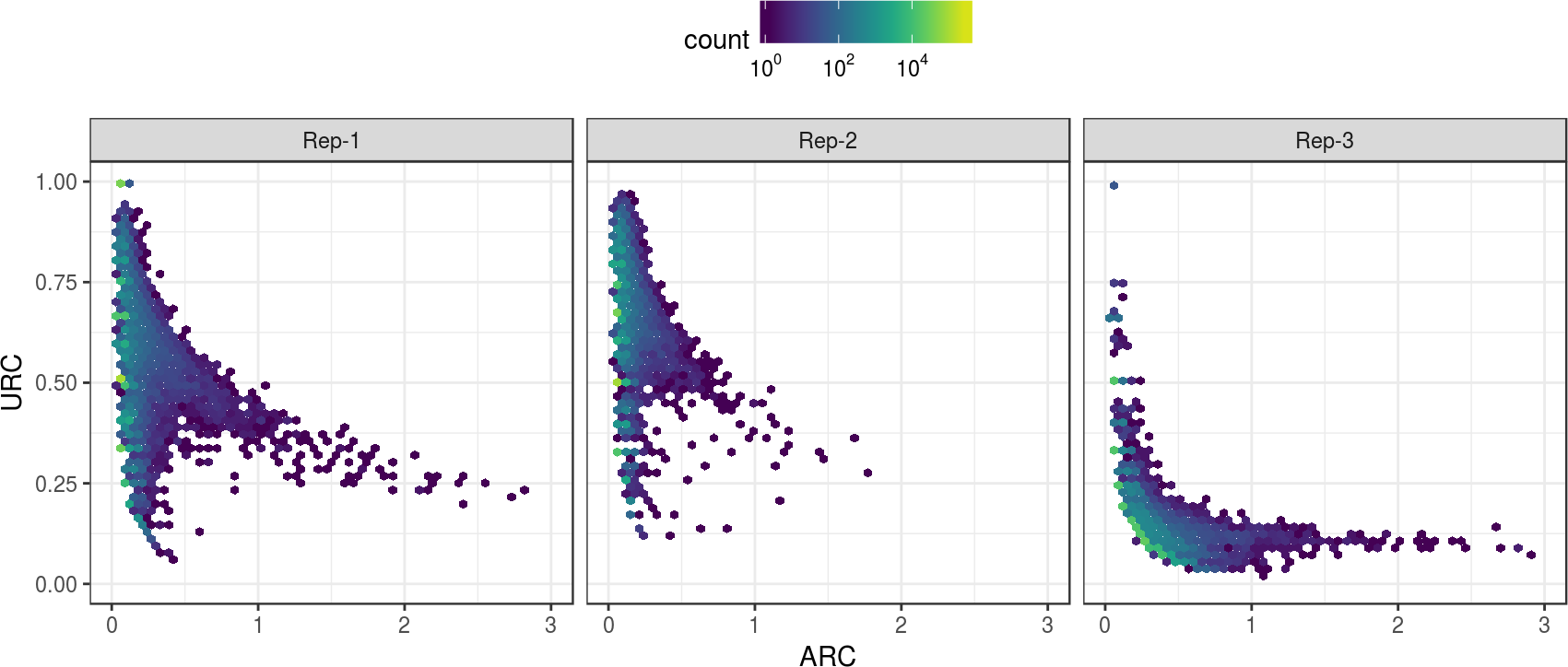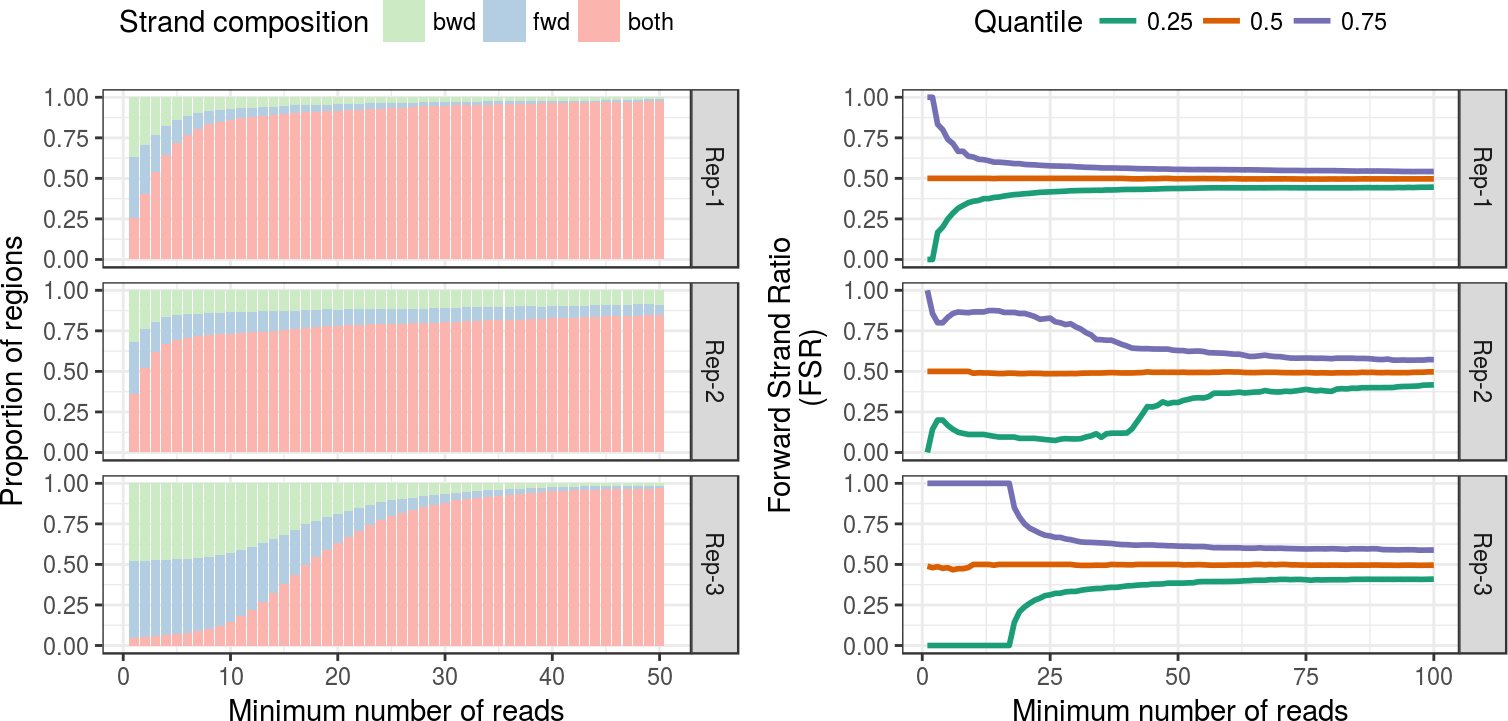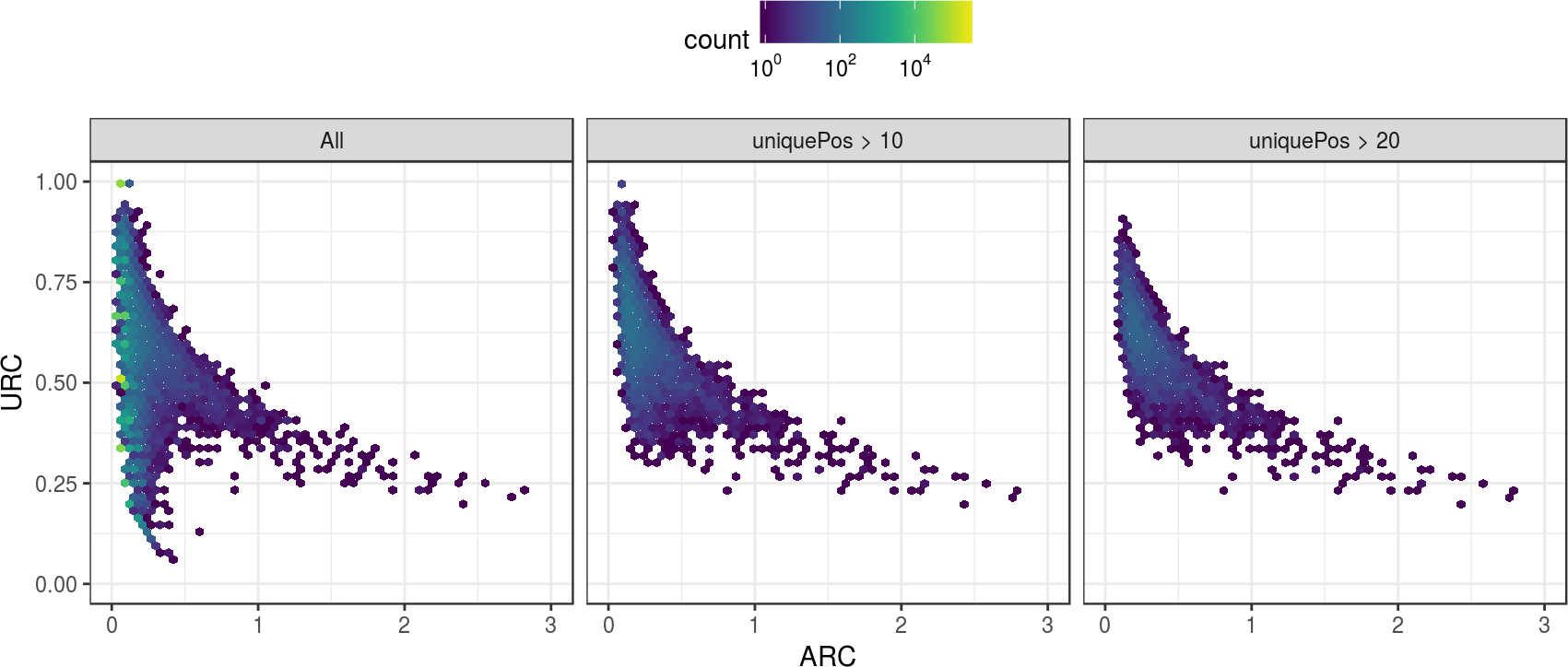# 1 Overview

In this vignette, we provide a brief overview of the ChIPexoQual package. This package provides a statistical quality control (QC) pipeline that enables the exploration and analysis of ChIP-exo/nexus experiments. In this vignette we used the reads aligned to chr1 in the mouse liver ChIP-exo experiment (Serandour et al. 2013) to illustrate the use of the pipeline. To load the packages we use:

    library(ChIPexoQual)
library(ChIPexoQualExample)

ChIPexoQual takes a set of aligned reads from a ChIP-exo (or ChIP-nexus) experiment as input and performs the following steps:

2. Compute $$D_i$$, number of reads in island $$i$$, and $$U_i$$, number of island $$i$$ positions with at least one aligning read, $$i=1, \cdots, I$$.
• For each island $$i$$, $$i=1, \cdots, I$$ compute island statistics: \begin{align*} \mbox{ARC}_i &= \frac{D_i}{W_i}, \quad \mbox{URC}_i = \frac{U_i}{D_i}, \\ %\mbox{URC}_i &= \frac{U_i}{D_i}, \\ \mbox{FSR}_i &= \frac{(\text{Number of forward strand reads aligning to island i})}{D_i}, \end{align*}
where $$W_i$$ denotes the width of island $$i$$,.
3. Generate diagnostic plots (i) URC vs. ARC plot; (ii) Region Composition plot; (iii) FSR distribution plot.
4. Randomly sample $$M$$ (at least 1000) islands and fit, \begin{align*} D_i = \beta_1 U_i + \beta_2 W_i + \varepsilon_i, \end{align*} where $$\varepsilon_i$$ denotes the independent error term. Repeat this process $$B$$ times and generate box plots of estimated $$\beta_1$$ and $$\beta_2$$.

We analyzed a larger collection of ChIP-exo/nexus experiments in (Welch et al. 2016) including complete versions of this samples.

# 2 Creating an ExoData object

The minimum input to use ChIPexoQual are the aligned reads of a ChIP-exo/nexus experiment. ChIPexoQual accepts either the name of the bam file or the reads in a GAlignments object:

    files = list.files(system.file("extdata",
package = "ChIPexoQualExample"),full.names = TRUE)
basename(files)
##  "ChIPexo_carroll_FoxA1_mouse_rep1_chr1.bam"
    ex1 = ExoData(file = files,mc.cores = 2L,verbose = FALSE)
ex1
## ExoData object with 655785 ranges and 11 metadata columns:
##               <Rle>              <IRanges>  <Rle> | <integer> <integer>
##             chr1     [3000941, 3000976]      * |         2         0
##             chr1     [3001457, 3001492]      * |         0         1
##             chr1     [3001583, 3001618]      * |         0         2
##             chr1     [3001647, 3001682]      * |         1         0
##             chr1     [3001852, 3001887]      * |         1         0
##        ...      ...                    ...    ... .       ...       ...
##        chr1 [197192012, 197192047]      * |         0         1
##        chr1 [197192421, 197192456]      * |         0         1
##        chr1 [197193059, 197193094]      * |         1         0
##        chr1 [197193694, 197193729]      * |         0         3
##        chr1 [197194986, 197195021]      * |         0         2
##               fwdPos    revPos     depth uniquePos                ARC
##            <integer> <integer> <integer> <integer>          <numeric>
##                 1         0         2         1 0.0555555555555556
##                 0         1         1         1 0.0277777777777778
##                 0         1         2         1 0.0555555555555556
##                 1         0         1         1 0.0277777777777778
##                 1         0         1         1 0.0277777777777778
##        ...       ...       ...       ...       ...                ...
##            0         1         1         1 0.0277777777777778
##            0         1         1         1 0.0277777777777778
##            1         0         1         1 0.0277777777777778
##            0         1         3         1 0.0833333333333333
##            0         1         2         1 0.0555555555555556
##                          URC       FSR         M         A
##                    <numeric> <numeric> <numeric> <numeric>
##                       0.5         1      -Inf       Inf
##                         1         0      -Inf      -Inf
##                       0.5         0      -Inf      -Inf
##                         1         1      -Inf       Inf
##                         1         1      -Inf       Inf
##        ...               ...       ...       ...       ...
##                    1         0      -Inf      -Inf
##                    1         0      -Inf      -Inf
##                    1         1      -Inf       Inf
##    0.333333333333333         0      -Inf      -Inf
##                  0.5         0      -Inf      -Inf
##   -------
##   seqinfo: 1 sequence from an unspecified genome; no seqlengths
    reads = readGAlignments(files,param = NULL)
identical(GRanges(ex1),GRanges(ex2))
##  TRUE

For the rest of the vignette, we generate an ExoData object for each replicate:

    files = files[grep("bai",files,invert = TRUE)] ## ignore index files
exampleExoData = lapply(files,ExoData,mc.cores = 2L,verbose = FALSE)

Finally, we can recover the number of reads that compose a ExoData object by using the nreads function:

    sapply(exampleExoData,nreads)
##  1654985 1766665 1670117

## 2.1 Enrichment analysis and library complexity:

To create the ARC vs URC plot proposed in (Welch et al. 2016), we use the ARC_URC_plot function. This function allows to visually compare different samples:

    ARCvURCplot(exampleExoData,names.input = paste("Rep",1:3,sep = "-"))This plot typically exhibits one of the following three patterns for any given sample. In all three panels we can observe two arms: the first with low Average Read Coefficient (ARC) and varying Unique Read Coefficient (URC); and the second where the URC decreases as the ARC increases. The first and third replicates exhibit a defined decreasing trend in URC as the ARC increases. This indicates that these samples exhibit a higher ChIP enrichment than the second replicate. On the other hand, the overall URC level from the first two replicates is higher than that of the third replicate, elucidating that the libraries for the first two replicates are more complex than that of the third replicate.

## 2.2 Strand imbalance

To create the FSR distribution and Region Composition plots suggested in Welch et. al 2016 (submitted), we use the FSR_dist_plot and region_comp_plot, respectively.

    p1 = regionCompplot(exampleExoData,names.input = paste("Rep",1:3,
sep = "-"),depth.values = seq_len(50))
p2 = FSRDistplot(exampleExoData,names.input = paste("Rep",1:3,sep = "-"),
quantiles = c(.25,.5,.75),depth.values = seq_len(100))
gridExtra::grid.arrange(p1,p2,nrow = 1)The left panel displays the Region Composition plot and the right panel shows the Forward Strand Ratio (FSR) distribution plot, both of which highlight specific problems with replicates 2 and 3. The Region Composition plot exhibits apparent decreasing trends in the proportions of regions formed by fragments in one exclusive strand. High quality experiments tend to show exponential decay in the proportion of single stranded regions, while for the lower quality experiments, the trend may be linear or even constant. The FSR distributions of both of replicates 2 and 3 are more spread around their respective medians. The rate at which the FSR distribution becomes more centralized around the median indicates the aforementioned lower enrichment in the second replicate and the low complexity in the third one. The asymmetric behavior of the second replicate is characteristic of low enrichment, while the constant values of replicate three for low minimum number of reads indicate that this replicate has islands composed of reads aligned to very few unique positions.

### 2.2.1 Further exploration of ChIP-exo data

All the plot functions in ChIPexoQual allow a list or several separate ExoData objects. This allows to explore island subsets for each replicate. For example, to show that the first arm is composed of regions formed by reads aligned to few positions, we can generate the following plot:

    ARCvURCplot(exampleExoData[],
subset(exampleExoData[],uniquePos > 10),
subset(exampleExoData[],uniquePos > 20),
names.input = c("All", "uniquePos > 10", "uniquePos > 20"))For this figure, we used the ARC vs URC plot to show how several of the regions with low ARC values are composed by reads that align to a small number of unique positions. This technique highlights a strategy that can be followed to further explore the data, as with all the previously listed plotting functions we may compare different subsets of the islands in the partition.

## 2.3 Quality evaluation

The last step of the quality control pipeline is to evaluate the linear model:

\begin{align*} D_i = \beta_1 U_i + \beta_2 U_2 + \epsilon_i, \end{align*}

The distribution of the parameters of this model is built by sampling nregions regions (the default value is 1,000), fitting the model and repeating the process ntimes (the default value is 100). We visualize the distributions of the parameters with box-plots:

    p1 = paramDistBoxplot(exampleExoData,which.param = "beta1", names.input = paste("Rep",1:3,sep = "-"))
p2 = paramDistBoxplot(exampleExoData,which.param = "beta2", names.input = paste("Rep",1:3,sep = "-"))
gridExtra::grid.arrange(p1,p2,nrow = 1)